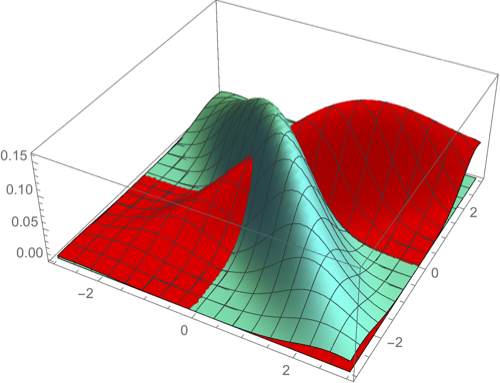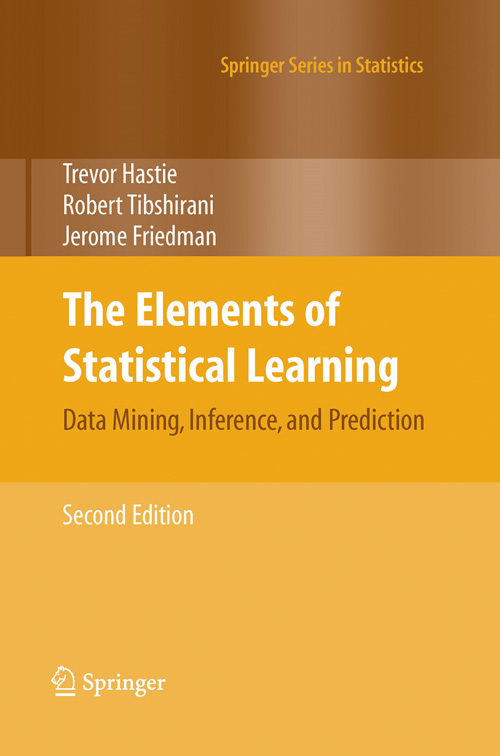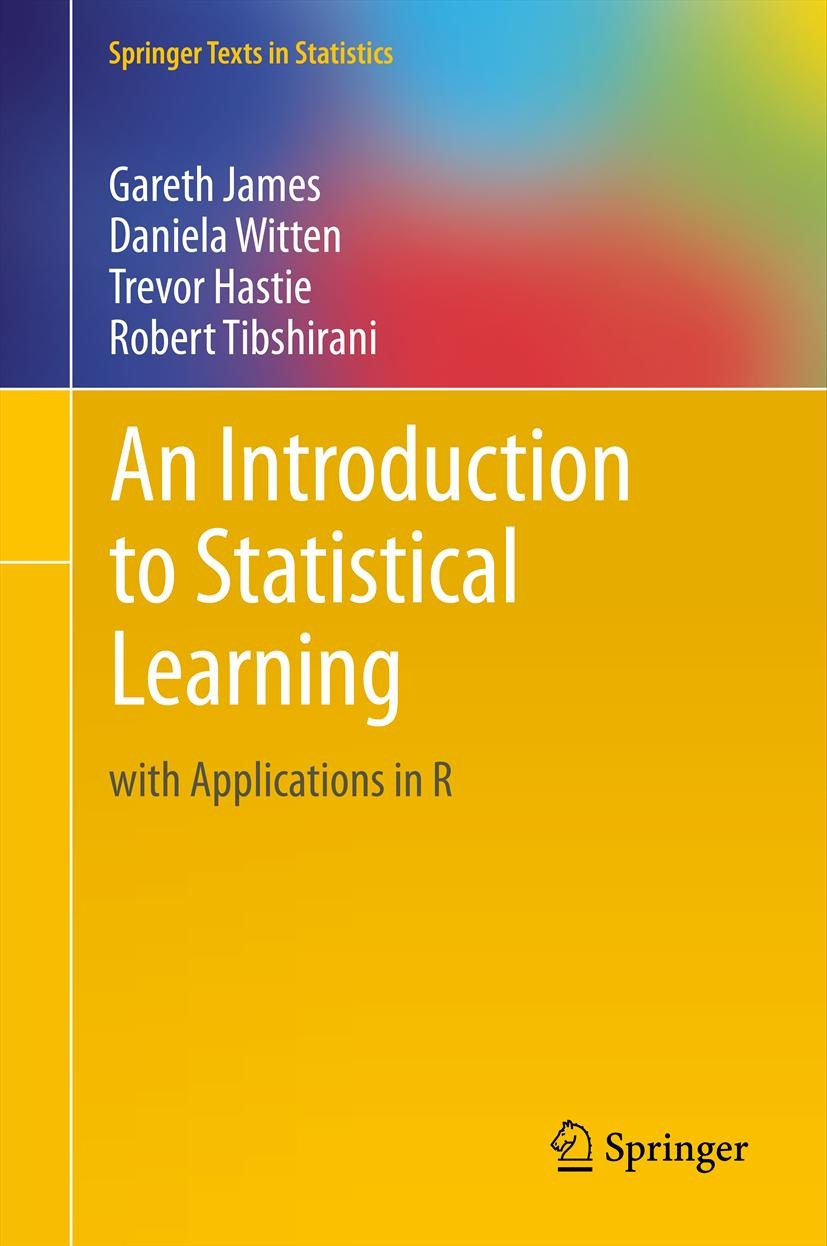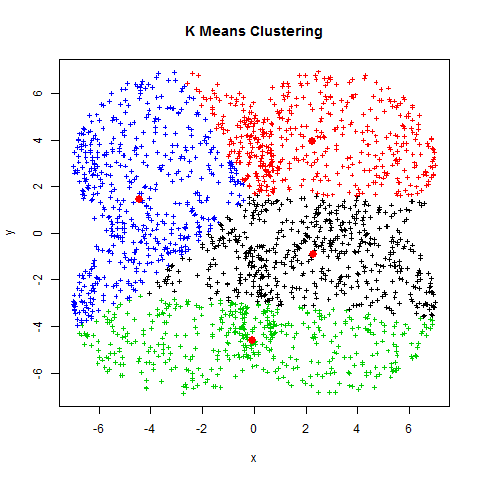CS 189/289A Introduction to Machine Learning Jonathan Shewchuk(Please send email only if you don't want anyone but me to see it; otherwise, use Piazza.) Spring 2019 Mondays and Wednesdays, 6:30–8:00 pm Wheeler Hall Auditorium (a.k.a. 150 Wheeler Hall) My office hours: Mondays, 5:10–6 pm, 529 Soda Hall, Wednesdays, 9:10–10 pm, 411 Soda Hall, and by appointment. (I'm usually free after the lectures too.)

This class introduces algorithms for learning, which constitute an important part of artificial intelligence.

Topics include

• classification: perceptrons, support vector machines (SVMs), Gaussian discriminant analysis (including linear discriminant analysis, LDA, and quadratic discriminant analysis, QDA), logistic regression, decision trees, neural networks, convolutional neural networks, boosting, nearest neighbor search;
• regression: least-squares linear regression, logistic regression, polynomial regression, ridge regression, Lasso;
• density estimation: maximum likelihood estimation (MLE);
• dimensionality reduction: principal components analysis (PCA), random projection, latent factor analysis; and
• clustering: k-means clustering, hierarchical clustering, spectral graph clustering.

### Prerequisites

• Math 53 (or another vector calculus course),
• Math 54, Math 110, or EE 16A+16B (or another linear algebra course),
• CS 70, EECS 126, or Stat 134 (or another probability course).
You should take these prerequisites quite seriously: if you don't have them, I strongly recommend not taking CS 189.

If you want to brush up on prerequisite material, Stanford's machine learning class provides nice reviews of linear algebra and probability theory. Here's a shorter summary of math for machine learning written by our former TA Garrett Thomas. There's a fantastic collection of linear algebra visualizations on YouTube by 3Blue1Brown starting with this playlist. Other suggestions for review material appear in this Piazza post.

### TextbooksBoth textbooks for this class are available free online. Hardcover and eTextbook versions are also available.

### Homework and Exams

You have a total of 5 slip days that you can apply to your semester's homework. We will simply not award points for any late homework you submit that would bring your total slip days over five.

Homework 1 is due Wednesday, January 30 at 11:59 PM. (Here's just the written part.)

Homework 2 is due Wednesday, February 13 at 11:59 PM. (It's just one PDF file. That's all.)

Homework 3 is due Wednesday, February 27 at 11:59 PM. (Here's just the written part.)

Homework 4 is due Wednesday, March 13 at 11:59 PM. (Here's just the written part.)

Homework 5 is due Wednesday, April 3 at 11:59 PM. (Here's just the written part.)

Homework 6 is due Friday, April 26 at 11:59 PM. (Here's just the written part.)

Homework 7 is due Wednesday, May 8 at 11:59 PM. (Here's just the written part.)

The CS 289A Project has a proposal due Monday, April 8. The video and final report are due Friday, May 10.

The Midterm took place on Monday, March 18 in class. Previous midterms are available: Without solutions: Spring 2013, Spring 2014, Spring 2015, Fall 2015, Spring 2016, Spring 2017, Spring 2019. With solutions: Spring 2013, Spring 2014, Spring 2015, Fall 2015, Spring 2016, Spring 2017, Spring 2019.

The Final Exam takes place on Friday, May 17, 3–6 PM. CS 189 is in exam group 19. Previous final exams are available. Without solutions: Spring 2013, Spring 2014, Spring 2015, Fall 2015, Spring 2016, Spring 2017. With solutions: Spring 2013, Spring 2014, Spring 2015, Fall 2015, Spring 2016, Spring 2017.

### Lectures

Lecture 1 (January 23): Introduction. Classification, training, and testing. Validation and overfitting. Read ESL, Chapter 1. My lecture notes (PDF). The screencast.

Lecture 2 (January 28): Linear classifiers. Decision functions and decision boundaries. The centroid method. Perceptrons. Read parts of the Wikipedia Perceptron page. Optional: Read ESL, Section 4.5–4.5.1. My lecture notes (PDF). The screencast.

Lecture 3 (January 30): Gradient descent, stochastic gradient descent, and the perceptron learning algorithm. Feature space versus weight space. The maximum margin classifier, aka hard-margin support vector machine (SVM). Read ISL, Section 9–9.1. My lecture notes (PDF). The screencast.

Lecture 4 (February 4): The support vector classifier, aka soft-margin support vector machine (SVM). Features and nonlinear decision boundaries. Read ESL, Section 12.2 up to and including the first paragraph of 12.2.1. My lecture notes (PDF). The screencast.

Lecture 5 (February 6): Machine learning abstractions: application/data, model, optimization problem, optimization algorithm. Common types of optimization problems: unconstrained, constrained (with equality constraints), linear programs, quadratic programs, convex programs. Optional: Read (selectively) the Wikipedia page on mathematical optimization. My lecture notes (PDF). The screencast.

Lecture 6 (February 11): Decision theory: the Bayes decision rule and optimal risk. Generative and discriminative models. Read ISL, Section 4.4.1. My lecture notes (PDF). The screencast.

Lecture 7 (February 13): Gaussian discriminant analysis, including quadratic discriminant analysis (QDA) and linear discriminant analysis (LDA). Maximum likelihood estimation (MLE) of the parameters of a statistical model. Fitting an isotropic Gaussian distribution to sample points. Read ISL, Section 4.4. Optional: Read (selectively) the Wikipedia page on maximum likelihood. My lecture notes (PDF). The screencast.

February 18 is Presidents' Day.

Lecture 8 (February 20): Eigenvectors, eigenvalues, and the eigendecomposition. The Spectral Theorem for symmetric real matrices. The quadratic form and ellipsoidal isosurfaces as an intuitive way of understanding symmetric matrices. Application to anisotropic normal distributions (aka Gaussians). Read Chuong Do's notes on the multivariate Gaussian distribution. My lecture notes (PDF). The screencast.

Lecture 9 (February 25): Anisotropic normal distributions (aka Gaussians). MLE, QDA, and LDA revisited for anisotropic Gaussians. Read ISL, Sections 4.4 and 4.5. My lecture notes (PDF). The screencast.

Lecture 10 (February 27): Regression: fitting curves to data. The 3-choice menu of regression function + loss function + cost function. Least-squares linear regression as quadratic minimization and as orthogonal projection onto the column space. The design matrix, the normal equations, the pseudoinverse, and the hat matrix (projection matrix). Logistic regression; how to compute it with gradient descent or stochastic gradient descent. Read ISL, Sections 4–4.3. My lecture notes (PDF). The screencast.

Lecture 11 (March 4): Newton's method and its application to logistic regression. LDA vs. logistic regression: advantages and disadvantages. ROC curves. Weighted least-squares regression. Least-squares polynomial regression. Read ISL, Sections 4.4.3, 7.1, 9.3.3; ESL, Section 4.4.1. Optional: here is a fine short discussion of ROC curves—but skip the incoherent question at the top and jump straight to the answer. My lecture notes (PDF). The screencast.

Lecture 12 (March 6): Statistical justifications for regression. The empirical distribution and empirical risk. How the principle of maximum likelihood motivates the cost functions for least-squares linear regression and logistic regression. The bias-variance decomposition; its relationship to underfitting and overfitting; its application to least-squares linear regression. Read ESL, Sections 2.5 and 2.9. Optional: Read the Wikipedia page on the bias-variance trade-off. My lecture notes (PDF). The screencast.

Lecture 13 (March 11): Ridge regression: penalized least-squares regression for reduced overfitting. How the principle of maximum a posteriori (MAP) motivates the penalty term (aka Tikhonov regularization). Subset selection. Lasso: penalized least-squares regression for reduced overfitting and subset selection. Read ISL, Sections 6–6.1.2, the last part of 6.1.3 on validation, and 6.2–6.2.1; and ESL, Sections 3.4–3.4.3. Optional: This CrossValidated page on ridge regression is pretty interesting. My lecture notes (PDF). The screencast.

Note: I have moved the lecture on kernels until after Spring Recess, so that the lectures on decision trees won't be split across the break. However, I'm still calling the lecture on kernels Lecture 14.

Lecture 15 (March 13): Decision trees; algorithms for building them. Entropy and information gain. Read ISL, Sections 8–8.1. My lecture notes (PDF). The screencast.

The Midterm takes place on Monday, March 18. You are permitted one “cheat sheet” of letter-sized (8½" × 11") paper.

Lecture 16 (March 20): More decision trees: multivariate splits; decision tree regression; stopping early; pruning. Ensemble learning: bagging (bootstrap aggregating), random forests. Read ISL, Section 8.2. My lecture notes (PDF). The screencast.

March 25–29 is Spring Recess.

Lecture 14 (April 1): Kernels. Kernel ridge regression. The polynomial kernel. Kernel perceptrons. Kernel logistic regression. The Gaussian kernel. Optional: Read ISL, Section 9.3.2 and ESL, Sections 12.3–12.3.1 if you're curious about kernel SVM. My lecture notes (PDF). The screencast.

Lecture 17 (April 3): Neural networks. Gradient descent and the backpropagation algorithm. Read ESL, Sections 11.3–11.4. Optional: I've heard positive recommendations for Welch Labs' video tutorial Neural Networks Demystified on YouTube. Also of special interest is this Javascript neural net demo that runs in your browser. Here's another derivation of backpropagation that some people have found helpful. My lecture notes (PDF). The screencast.

Lecture 18 (April 8): Neuron biology: axons, dendrites, synapses, action potentials. Differences between traditional computational models and neuronal computational models. Backpropagation with softmax outputs and logistic loss. Unit saturation, aka the vanishing gradient problem, and ways to mitigate it. Heuristics for avoiding bad local minima. Optional: Try out some of the Javascript demos on this excellent web page—and if time permits, read the text too. The first four demos illustrate the neuron saturation problem and its fix with the logistic loss (cross-entropy) functions. The fifth demo gives you sliders so you can understand how softmax works. My lecture notes (PDF). The screencast.

Lecture 19 (April 10): Heuristics for faster training. Heuristics for avoiding bad local minima. Heuristics to avoid overfitting. Convolutional neural networks. Neurology of retinal ganglion cells in the eye and simple and complex cells in the V1 visual cortex. Read ESL, Sections 11.5 and 11.7. Here is the video about Hubel and Wiesel's experiments on the feline V1 visual cortex. Optional: A fine paper on heuristics for better neural network learning is Yann LeCun, Leon Bottou, Genevieve B. Orr, and Klaus-Robert Müller, “Efficient BackProp,” in G. Orr and K.-R. Müller (Eds.), Neural Networks: Tricks of the Trade, Springer, 1998. Also of special interest is this Javascript convolutional neural net demo that runs in your browser. My lecture notes (PDF). Some slides about the V1 visual cortex and ConvNets (PDF). The screencast.

Lecture 20 (April 15): Unsupervised learning. Principal components analysis (PCA). Derivations from maximum likelihood estimation, maximizing the variance, and minimizing the sum of squared projection errors. Eigenfaces for face recognition. Read ISL, Sections 10–10.2 and the Wikipedia page on Eigenface. My lecture notes (PDF). The screencast.

Lecture 21 (April 17): The singular value decomposition (SVD) and its application to PCA. Clustering: k-means clustering aka Lloyd's algorithm; k-medoids clustering; hierarchical clustering; greedy agglomerative clustering. Dendrograms. Read ISL, Section 10.3. My lecture notes (PDF). The screencast.

Lecture 22 (April 22): Spectral graph partitioning and graph clustering. Relaxing a discrete optimization problem to a continuous one. The Fiedler vector, the sweep cut, and Cheeger's inequality. The vibration analogy. Greedy divisive clustering. The normalized cut and image segmentation. Read my survey of Spectral and Isoperimetric Graph Partitioning, Sections 1.2–1.4, 2.1, 2.2, 2.4, 2.5, and optionally A and E.2. For reference: Jianbo Shi and Jitendra Malik, Normalized Cuts and Image Segmentation, IEEE Transactions on Pattern Analysis and Machine Intelligence 22(8):888–905, 2000. My lecture notes (PDF). The screencast.

Lecture 23 (April 24): Graph clustering with multiple eigenvectors. Random projection. Latent factor analysis (aka latent semantic indexing). The geometry of high-dimensional spaces. Optional: Read the Wikipedia page on latent semantic analysis. Optional: Section E.2 of my survey. For reference: Andrew Y. Ng, Michael I. Jordan, and Yair Weiss, On Spectral Clustering: Analysis and an Algorithm (PostScript format), Advances in Neural Information Processing Systems 14 (Thomas G. Dietterich, Suzanna Becker, and Zoubin Ghahramani, editors), pages 849–856, the MIT Press, September 2002. For reference: Sanjoy Dasgupta and Anupam Gupta, An Elementary Proof of a Theorem of Johnson and Lindenstrauss, Random Structures and Algorithms 22(1)60–65, January 2003. My lecture notes (PDF). The screencast.

Lecture 24 (April 29): AdaBoost, a boosting method for ensemble learning. Nearest neighbor classification and its relationship to the Bayes risk. Read ESL, Sections 10–10.5, and ISL, Section 2.2.3. For reference: Yoav Freund and Robert E. Schapire, A Decision-Theoretic Generalization of On-Line Learning and an Application to Boosting, Journal of Computer and System Sciences 55(1):119–139, August 1997. My lecture notes (PDF). The screencast.

Lecture 25 (May 1): The exhaustive algorithm for k-nearest neighbor queries. Speeding up nearest neighbor queries. Voronoi diagrams and point location. k-d trees. Application of nearest neighbor search to the problem of geolocalization: given a query photograph, determine where in the world it was taken. If I like machine learning, what other classes should I take? For reference: the best paper I know about how to implement a k-d tree is Sunil Arya and David M. Mount, Algorithms for Fast Vector Quantization, Data Compression Conference, pages 381–390, March 1993. For reference: the IM2GPS web page, which includes a link to the paper. My lecture notes (PDF). The screencast.

The Final Exam takes place on Friday, May 17, 3–6 PM in Wheeler Hall Auditorium, a.k.a. 150 Wheeler Hall. (CS 189 is in exam group 19.)

### Discussion Sections and Teaching Assistants

Sections begin to meet on January 29. A schedule will appear here.

Office hours are listed here. If you're planning to visit, please consider filling out this online office hour queue form so your TA can prepare, and so you'll know how many other students to expect there.Supported in part by the National Science Foundation under Awards CCF-0430065, CCF-0635381, IIS-0915462, and CCF-1423560, in part by a gift from the Okawa Foundation, and in part by an Alfred P. Sloan Research Fellowship.